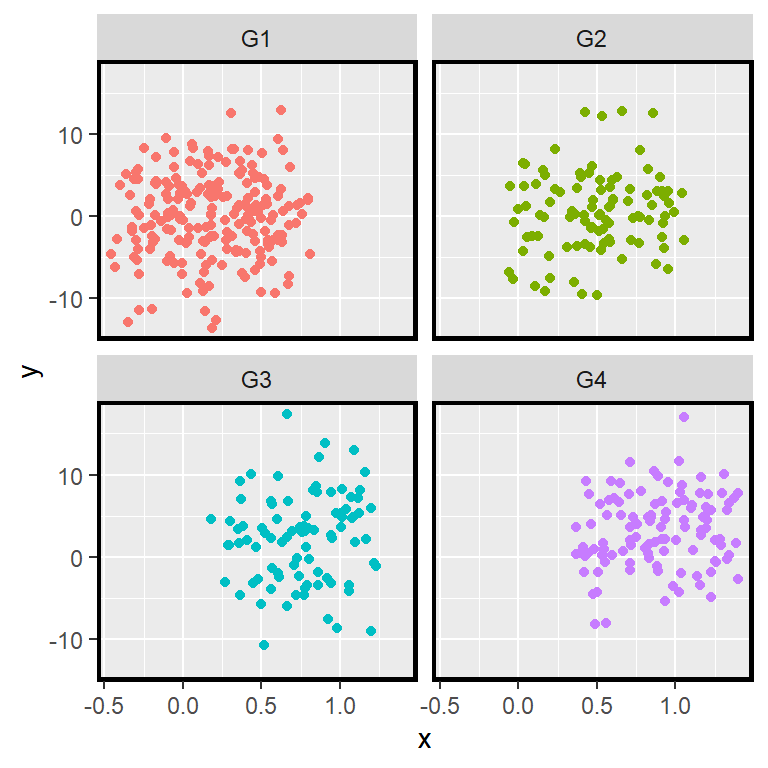# Faceting in ggplot2 with facet_wrap and facet_grid

When using ggplot2 you can create multi panel plots, also known as Trellis plots or facets with the `facet_grid` or `facet_wrap` functions. These functions are similar, but there are some differences between them, as the former creates a matrix of panels based on two discrete variables (it also works with one, but its not recommended) while the latter creates a ribbon of plots based on a single discrete variable (it also works with two, but its not recommended).

## One discrete variable: `facet_wrap`

Consider, for instance, that you have a set of observations that belong to different groups. In this scenario, you can display them in the same graph and highlight the groups with a different color or marker.

``````# install.packages("ggplot2")
library(ggplot2)

# Data simulation
set.seed(4)
x <- runif(500)
y <- 4 * x ^ 2 + rnorm(length(x), sd = 5)
group <- ifelse(x < 0.4, "G1", ifelse(x < 0.6, "G2", ifelse(x < 0.8, "G3", "G4")))
x <- x + runif(length(x), -0.5, 0.5)

# Data frame
df <- data.frame(x = x, y = y, group = group)

# Scatter plot by group
ggplot(df, aes(x = x, y = y, color = group)) +
geom_point()``````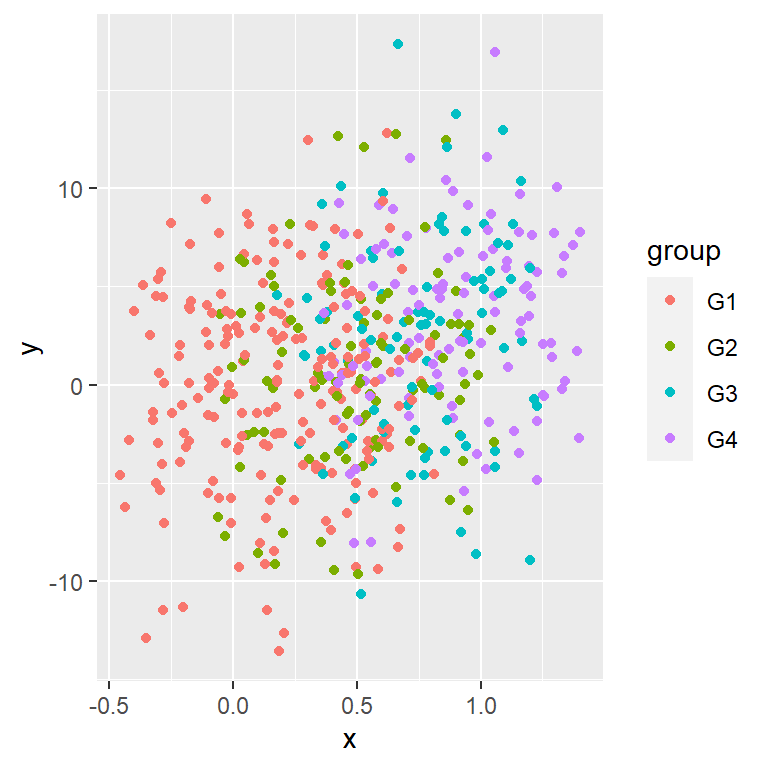The problem is that sometimes the chart is not readable, so an alternative is to split the data into different plots to see each group individually. For this purpose you can make use of the `facet_wrap` function passing the grouping variable as formula, e.g. `~group`.

``````# install.packages("ggplot2")
library(ggplot2)

# Data simulation
set.seed(4)
x <- runif(500)
y <- 4 * x ^ 2 + rnorm(length(x), sd = 5)
group <- ifelse(x < 0.4, "G1", ifelse(x < 0.6, "G2", ifelse(x < 0.8, "G3", "G4")))
x <- x + runif(length(x), -0.5, 0.5)

# Data frame
df <- data.frame(x = x, y = y, group = group)

# Ribbon of plots
ggplot(df, aes(x = x, y = y, color = group)) +
geom_point(show.legend = FALSE) +
facet_wrap(~group)``````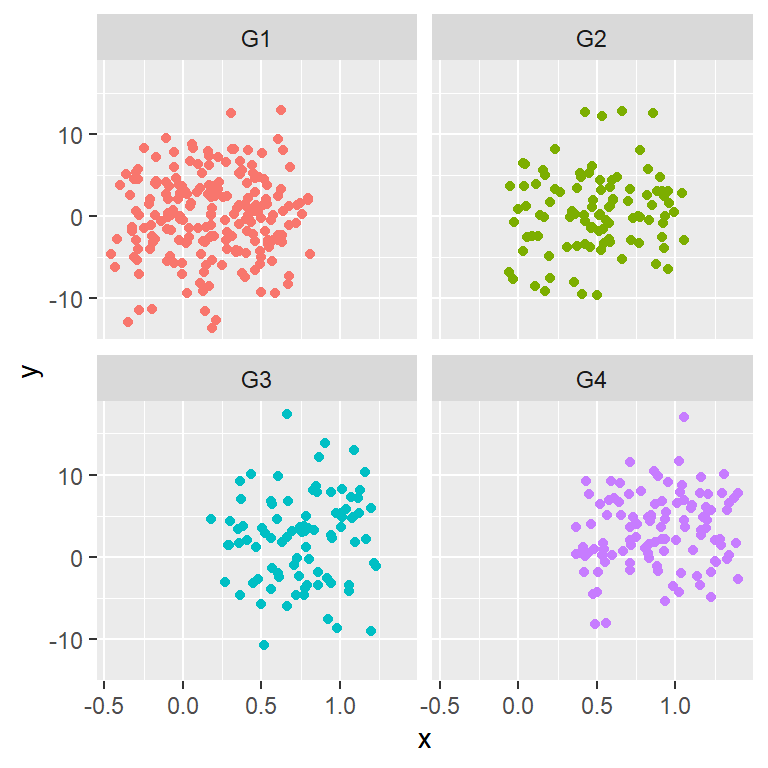Change the order of the panels ordering the levels of the categorical variable with `factor`.

Number of rows and columns

The default number of rows and columns will depend on your data. If your categorical variable has four groups the `facet_wrap` function will create two columns and two rows, but in this example maybe having only one row is more readable, so you can set `nrow = 1` or `ncol = 4`.

``````# install.packages("ggplot2")
library(ggplot2)

# Data simulation
set.seed(4)
x <- runif(500)
y <- 4 * x ^ 2 + rnorm(length(x), sd = 5)
group <- ifelse(x < 0.4, "G1", ifelse(x < 0.6, "G2", ifelse(x < 0.8, "G3", "G4")))
x <- x + runif(length(x), -0.5, 0.5)

# Data frame
df <- data.frame(x = x, y = y, group = group)

# Ribbon of plots (2 columns)
ggplot(df, aes(x = x, y = y, color = group)) +
geom_point(show.legend = FALSE) +
facet_wrap(~group, nrow = 1) # Or ncol= 4``````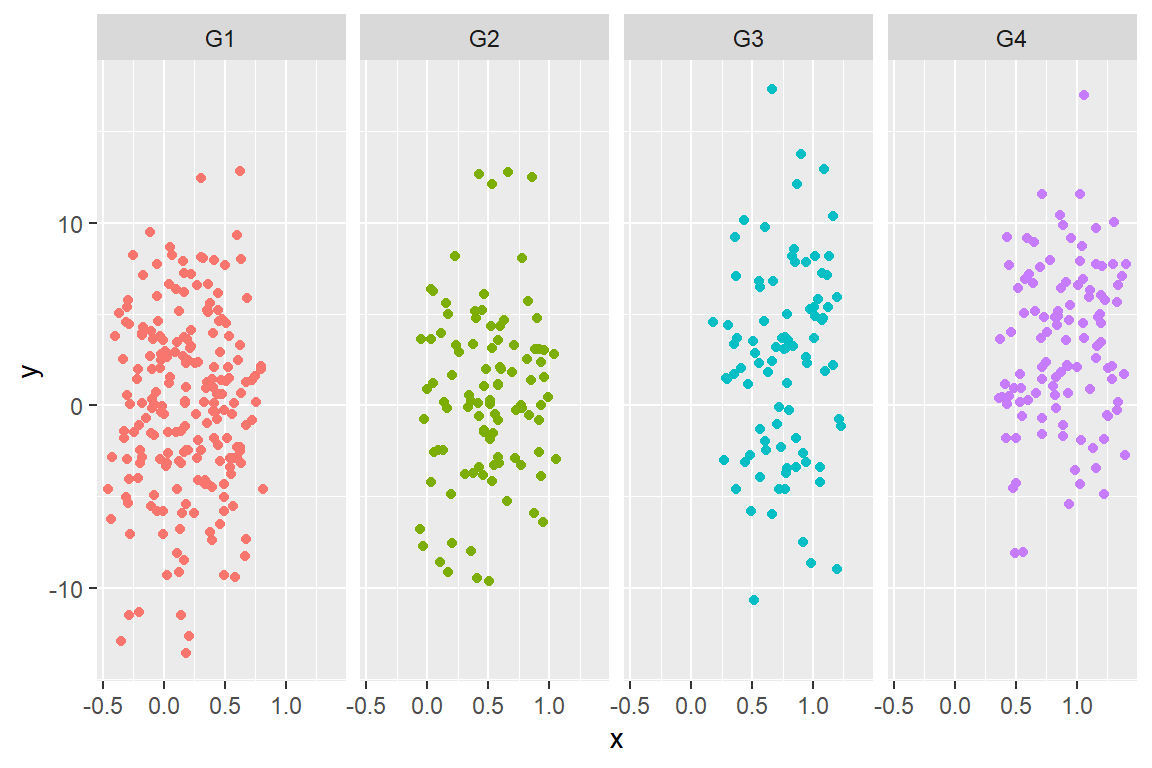If instead of `facet_wrap` we use `facet_grid`, the same panels will be created, but there would always be only one row or only one column. That’s why its use its not recommended and is better to use `facet_wrap` and be able to choose the number of rows or columns.

Axis scales

By default, all the panels will have the same scale along the axis, but when the data is in different scale you can set `scales = "free"`, so each plot will have a different range. Setting the scales free makes easier to see patterns on each panel, while fixed makes easier to find patterns across panels. Note that you can also free a single axis with `scales = "free_x"` or `scales = "free_y"`.

``````# install.packages("ggplot2")
library(ggplot2)

# Data simulation
set.seed(4)
x <- runif(500)
y <- 4 * x ^ 2 + rnorm(length(x), sd = 5)
group <- ifelse(x < 0.4, "G1", ifelse(x < 0.6, "G2", ifelse(x < 0.8, "G3", "G4")))
x <- x + runif(length(x), -0.5, 0.5)

# Data frame
df <- data.frame(x = x, y = y, group = group)

# Ribbon of plots
ggplot(df, aes(x = x, y = y, color = group)) +
geom_point(show.legend = FALSE) +
facet_wrap(~group, scales = "free")``````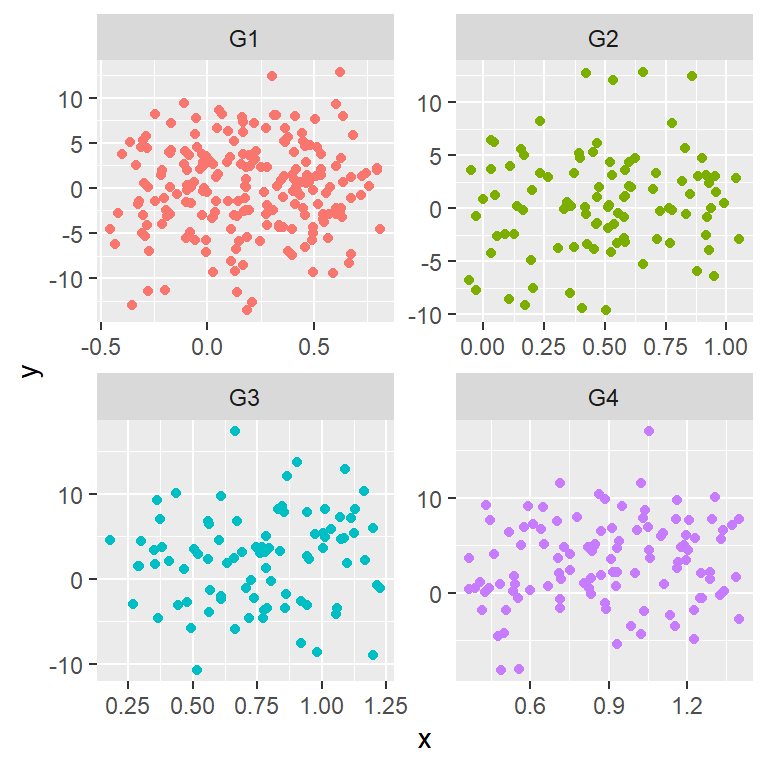Panels direction

The default direction for the panels is horizontal (`dir = "h"`) but you can also set it to vertical with `dir = "v"`. See that the upper right panel now corresponds to the third groups instead to the second.

``````# install.packages("ggplot2")
library(ggplot2)

# Data simulation
set.seed(4)
x <- runif(500)
y <- 4 * x ^ 2 + rnorm(length(x), sd = 5)
group <- ifelse(x < 0.4, "G1", ifelse(x < 0.6, "G2", ifelse(x < 0.8, "G3", "G4")))
x <- x + runif(length(x), -0.5, 0.5)

# Data frame
df <- data.frame(x = x, y = y, group = group)

# Vertical ribbon of plots
ggplot(df, aes(x = x, y = y, color = group)) +
geom_point(show.legend = FALSE) +
facet_wrap(~group, dir = "v")``````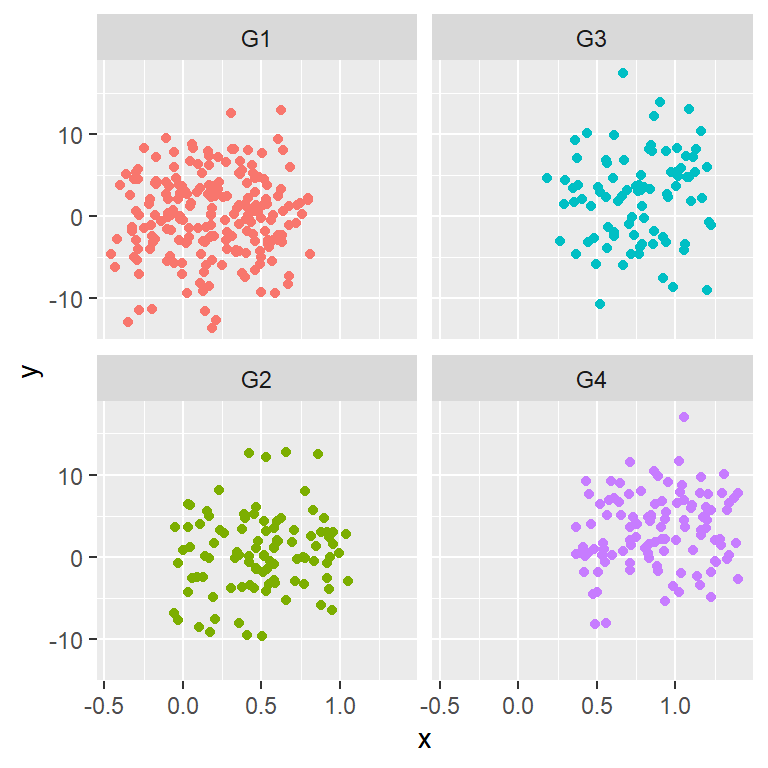Labels position

The `facet_wrap` function provides an argument named `strip.position` that allows customizing the position of the labels. The default value is `"top"`, but it could also be `"bottom"`, `"left"` or `"right"`.

``````# install.packages("ggplot2")
library(ggplot2)

# Data simulation
set.seed(4)
x <- runif(500)
y <- 4 * x ^ 2 + rnorm(length(x), sd = 5)
group <- ifelse(x < 0.4, "G1", ifelse(x < 0.6, "G2", ifelse(x < 0.8, "G3", "G4")))
x <- x + runif(length(x), -0.5, 0.5)

# Data frame
df <- data.frame(x = x, y = y, group = group)

# Ribbon of plots
ggplot(df, aes(x = x, y = y, color = group)) +
geom_point(show.legend = FALSE) +
facet_wrap(~group, strip.position = "bottom")``````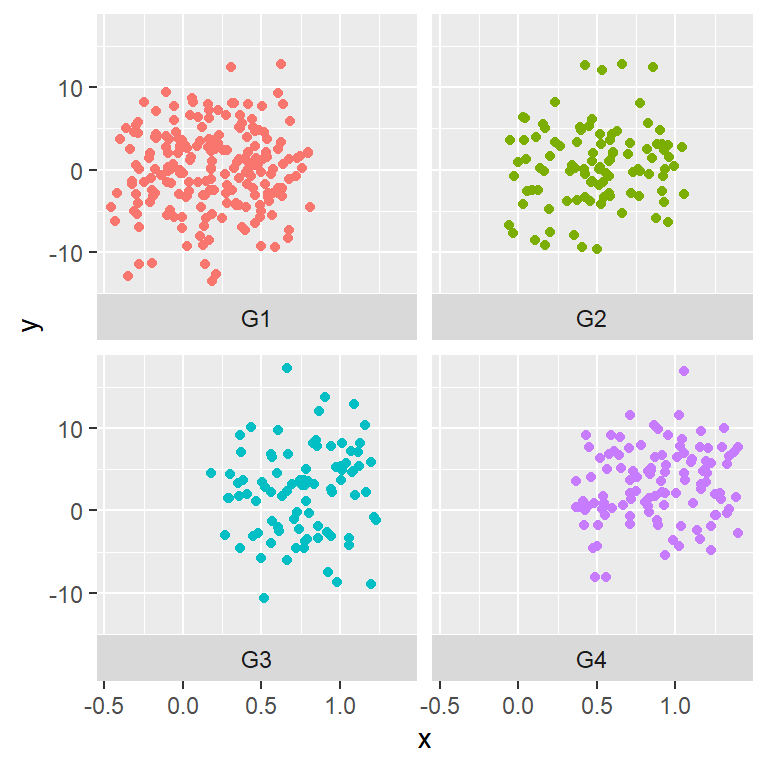Highlight each group showing all the data behind

It is possible to add all the data to all panels and highlight the corresponding data for each one. For this purpose you will need to create a new layer passing as input the same data frame used before but removing the categorical variable (in this example is named `group`).

``````# install.packages("ggplot2")
library(ggplot2)

# Data simulation
set.seed(4)
x <- runif(500)
y <- 4 * x ^ 2 + rnorm(length(x), sd = 5)
group <- ifelse(x < 0.4, "G1", ifelse(x < 0.6, "G2", ifelse(x < 0.8, "G3", "G4")))
x <- x + runif(length(x), -0.5, 0.5)

# Data frame
df <- data.frame(x = x, y = y, group = group)

# Ribbon of plots
ggplot(df, aes(x = x, y = y, color = group)) +
geom_point(data = transform(df, group = NULL), colour = "grey85") +
geom_point(show.legend = FALSE) +
facet_wrap(~group, strip.position = "bottom")``````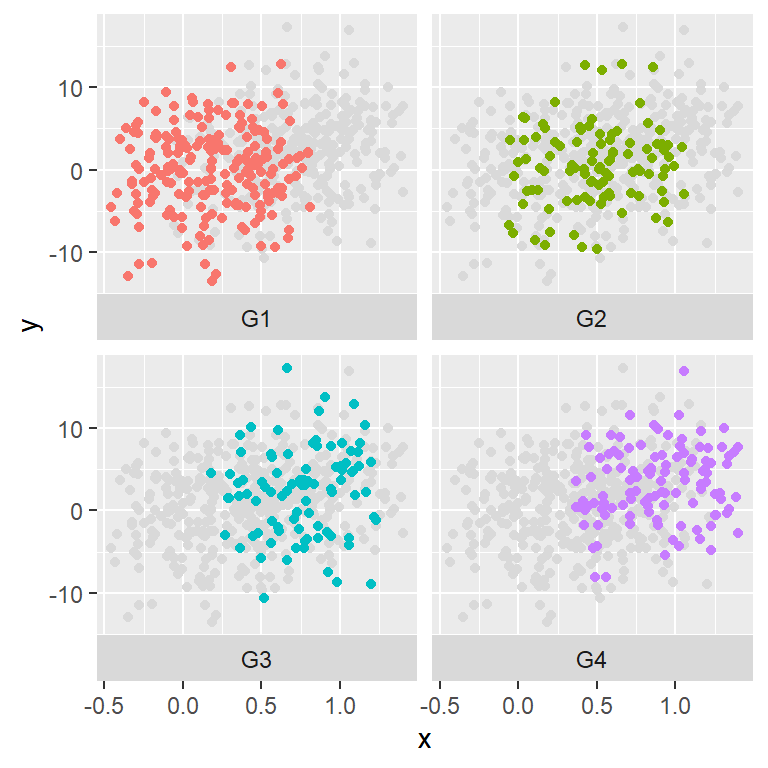## Two discrete variables: `facet_grid`

The `facet_grid` function is very similar to `facet_wrap`, but this function creates panels showing all the combinations of the data for two categorical variables. There are two ways to input data: the function can take a formula with the names of the categorical variables or you can pass the names of the variables to `rows` and `cols` arguments making use of the `vars` function.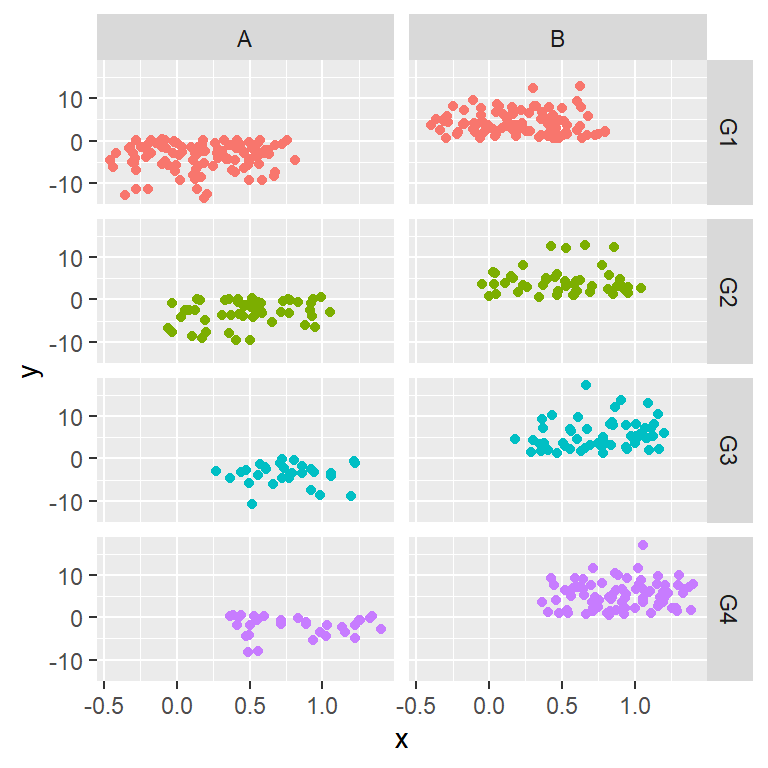``````# install.packages("ggplot2")
library(ggplot2)

# Data simulation
set.seed(4)
x <- runif(500)
y <- 4 * x ^ 2 + rnorm(length(x), sd = 5)
group1 <- ifelse(x < 0.4, "G1", ifelse(x < 0.6, "G2", ifelse(x < 0.8, "G3", "G4")))
group2 <- ifelse(y < 0.5, "A", "B")
x <- x + runif(length(x), -0.5, 0.5)

# Data frame
df <- data.frame(x = x, y = y, group1 = group1, group2 = group2)

# Matrix of plots
ggplot(df, aes(x = x, y = y, color = group1)) +
geom_point(show.legend = FALSE) +
facet_grid(group1 ~ group2) # Or facet_grid(rows = vars(group1), cols = vars(group2)) ``````

Axis scales

The `facet_grid` function also provides the `scales` argument to let the axis scales vary across rows and/or columns.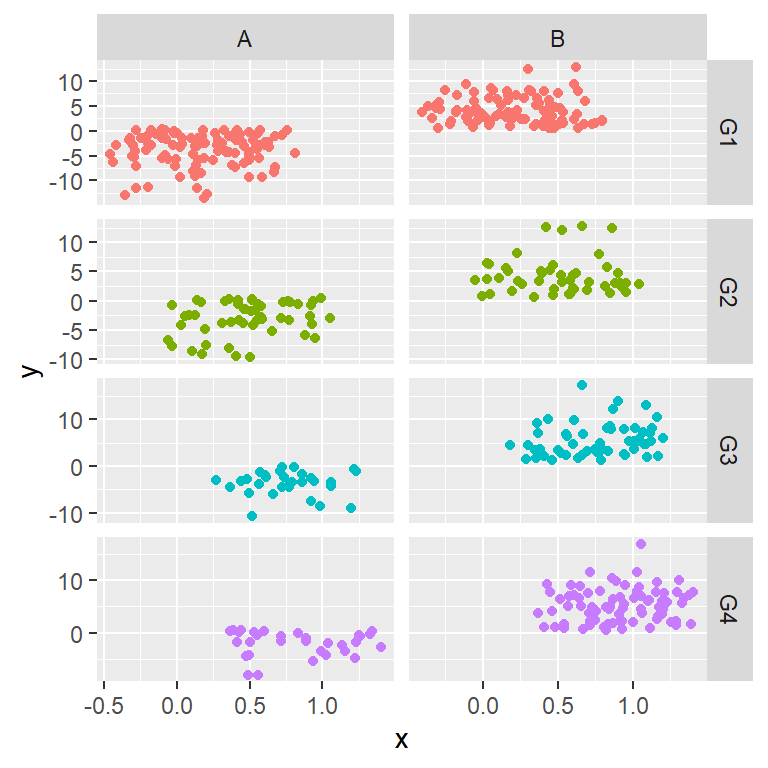``````# install.packages("ggplot2")
library(ggplot2)

# Data simulation
set.seed(4)
x <- runif(500)
y <- 4 * x ^ 2 + rnorm(length(x), sd = 5)
group1 <- ifelse(x < 0.4, "G1", ifelse(x < 0.6, "G2", ifelse(x < 0.8, "G3", "G4")))
group2 <- ifelse(y < 0.5, "A", "B")
x <- x + runif(length(x), -0.5, 0.5)

# Data frame
df <- data.frame(x = x, y = y, group1 = group1, group2 = group2)

# Matrix of plots
ggplot(df, aes(x = x, y = y, color = group1)) +
geom_point(show.legend = FALSE) +
facet_grid(group1 ~ group2, scales = "free")``````

Panels size

If the panels scales vary, you can use the `space` argument to make the panels have different size. Possible values are `"fixed"` (default), `"free_y"` to free the heights, `"free_x"`, to free the widths and `"free"` to let both the width and height vary. Note that in this example the argument doesn’t make any difference as all panels have the same scale.``````# install.packages("ggplot2")
library(ggplot2)

# Data simulation
set.seed(4)
x <- runif(500)
y <- 4 * x ^ 2 + rnorm(length(x), sd = 5)
group1 <- ifelse(x < 0.4, "G1", ifelse(x < 0.6, "G2", ifelse(x < 0.8, "G3", "G4")))
group2 <- ifelse(y < 0.5, "A", "B")
x <- x + runif(length(x), -0.5, 0.5)

# Data frame
df <- data.frame(x = x, y = y, group1 = group1, group2 = group2)

# Matrix of plots
ggplot(df, aes(x = x, y = y, color = group1)) +
geom_point(show.legend = FALSE) +
facet_grid(group1 ~ group2, space = "free_x")``````

The `facet_grid` function provides more arguments, such as `shrink`, `labeller`, `as.table`, `switch`, `drop` and `margins`. Recall to read the documentation for further details if needed.

## Further customization of the strips and panels

Using the arguments of the `theme` function you can customize the strips and panels. In the following examples we are going to review some use cases.

Customization of the strips of `facet_wrap`

You can customize the text styling of the labels with the `strip_text` argument. This argument takes the `element_text` function as input, where you can specify the different styles, such as the color, size, adjustment, etc. If you want to remove the labels use `element_blank` instead.

In order to customize the background color you will need to pass the `element_rect` function to `strip.background` and set the fill color with `fill`. In case you want to add a border you will need to set a line type, a color and a line width.

``````# install.packages("ggplot2")
library(ggplot2)

# Data simulation
set.seed(4)
x <- runif(500)
y <- 4 * x ^ 2 + rnorm(length(x), sd = 5)
group <- ifelse(x < 0.4, "G1", ifelse(x < 0.6, "G2", ifelse(x < 0.8, "G3", "G4")))
x <- x + runif(length(x), -0.5, 0.5)

# Data frame
df <- data.frame(x = x, y = y, group = group)

# Ribbon of plots
ggplot(df, aes(x = x, y = y, color = group)) +
geom_point(show.legend = FALSE) +
facet_wrap(~group) +
theme(strip.text = element_text(face = "bold", color = "white", hjust = 0, size = 15),
strip.background = element_rect(fill = "dodgerblue3", linetype = "solid",
color = "black", linewidth = 1))``````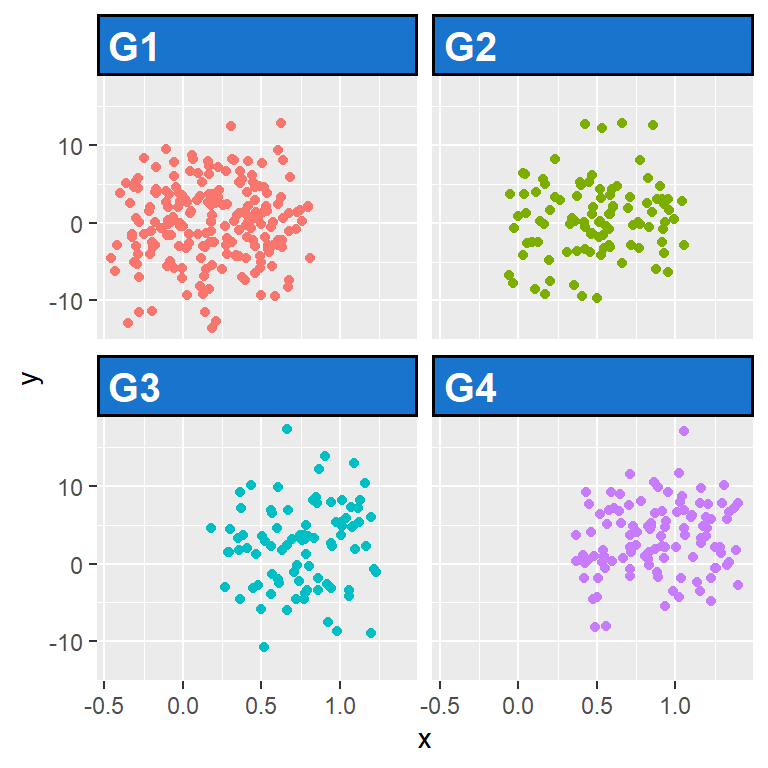Customization of the strips of `facet_grid`

If you have created a multi panel plot with `facet_grid` you can customize the strips the same way as in the example before, but you can also customize the labels for each axis individually making use of `strip.text.x`, `strip.text.y`, `strip.background.x` and `strip.background.y`.

``````library(ggplot2)

# Data simulation
set.seed(4)
x <- runif(500)
y <- 4 * x ^ 2 + rnorm(length(x), sd = 5)
group1 <- ifelse(x < 0.4, "G1", ifelse(x < 0.6, "G2", ifelse(x < 0.8, "G3", "G4")))
group2 <- ifelse(y < 0.5, "A", "B")
x <- x + runif(length(x), -0.5, 0.5)

# Data frame
df <- data.frame(x = x, y = y, group1 = group1, group2 = group2)

# Ribbon of plots
ggplot(df, aes(x = x, y = y,)) +
geom_point(show.legend = FALSE) +
facet_grid(group1 ~ group2) +
theme(strip.text.x = element_text(face = "bold", color = "white", hjust = 1, size = 15),
strip.text.y = element_text(face = "bold", color = "white", hjust = 0, size = 15),
strip.background.x = element_rect(fill = "dodgerblue3", linetype = "solid",
color = "black", linewidth = 1),
strip.background.y = element_rect(fill = "firebrick2", linetype = "solid",
color = "gray30", linewidth = 1))``````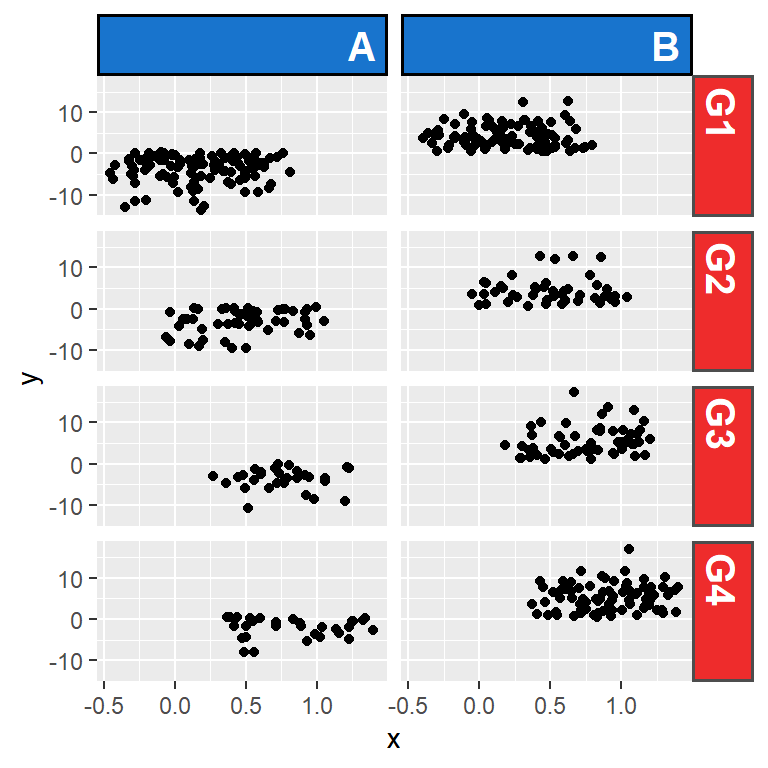Remove the background color of the strips

Note that it is possible to remove the background of the strips passing the `element_blank` function to `strip.background`.

``````# install.packages("ggplot2")
library(ggplot2)

# Data simulation
set.seed(4)
x <- runif(500)
y <- 4 * x ^ 2 + rnorm(length(x), sd = 5)
group <- ifelse(x < 0.4, "G1", ifelse(x < 0.6, "G2", ifelse(x < 0.8, "G3", "G4")))
x <- x + runif(length(x), -0.5, 0.5)

# Data frame
df <- data.frame(x = x, y = y, group = group)

# Ribbon of plots
ggplot(df, aes(x = x, y = y, color = group)) +
geom_point(show.legend = FALSE) +
facet_wrap(~group) +
theme(strip.text = element_text(size = 15),
strip.background = element_blank())``````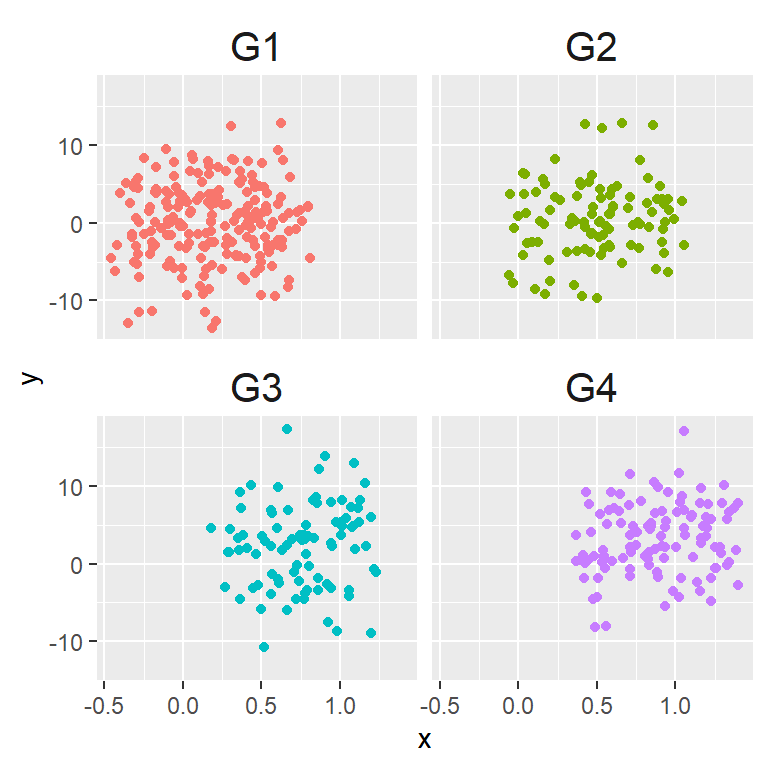Increase or remove the space between the panels

By default, there is a small space between the panels. You can increase or decrease that space passing a unit to the `panel.spacing` argument of the `theme` function.

``````# install.packages("ggplot2")
library(ggplot2)

# Data simulation
set.seed(4)
x <- runif(500)
y <- 4 * x ^ 2 + rnorm(length(x), sd = 5)
group <- ifelse(x < 0.4, "G1", ifelse(x < 0.6, "G2", ifelse(x < 0.8, "G3", "G4")))
x <- x + runif(length(x), -0.5, 0.5)

# Data frame
df <- data.frame(x = x, y = y, group = group)

# Ribbon of plots
ggplot(df, aes(x = x, y = y, color = group)) +
geom_point(show.legend = FALSE) +
facet_wrap(~group) +
theme(panel.spacing = unit(0, 'points'))``````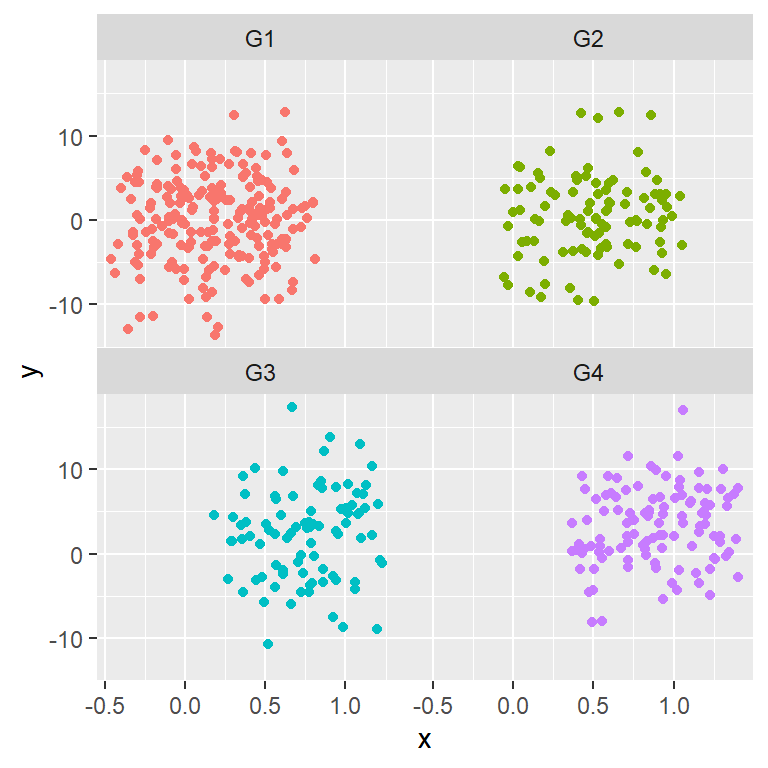Add a border to the panels

Recall that you can also customize other parameters of the plots, such as adding a border to the panels. You can achieve this with `panel.border`, following the example below.

``````library(ggplot2)

# Data simulation
set.seed(4)
x <- runif(500)
y <- 4 * x ^ 2 + rnorm(length(x), sd = 5)
group <- ifelse(x < 0.4, "G1", ifelse(x < 0.6, "G2", ifelse(x < 0.8, "G3", "G4")))
x <- x + runif(length(x), -0.5, 0.5)

# Data frame
df <- data.frame(x = x, y = y, group = group)

# Ribbon of plots
ggplot(df, aes(x = x, y = y, color = group)) +
geom_point(show.legend = FALSE) +
facet_wrap(~group) +
theme(panel.border = element_rect(fill = "transparent", # Needed to add the border
color = "black", linewidth = 1.5))``````